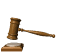"HydroCAD  University" .### TEL:  972-3-535 7602  972-3-534 7976 FAX: 972-3-534 3049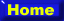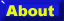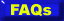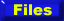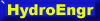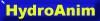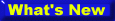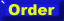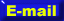What is Distance Learning ?

1) You get One lesson week by E-Mail , introducing the Subject by Text , Pictures , Equations , Analisys and Animation.
2) You may ask questions as much as you like .
3) You get some questions related to last lesson before starting the next subject .
4) You get "HydroCAd's University" expert evaluation .
5) Passing succesfuly all twelve subject you get a cetificate .

Syllabus.(Next -1 June , 2000)
1  )  Introduction to Hydraulic Transmissions. (1 lesson).
2  )  Hydraulic Pumps.(2 lessons).
3  ) Hydraulic Motors. (2 lessons).
4  ) Hydraulic Actuators.(2 lessons).
5  ) Hydraulic Logic Valves.(2 lessons).
6  ) Hydraulic Pressure Control Valves.(2 lessons).
7  ) Hydraulic Flow Control Valves.(2 lessons).
8  ) Hydraulic Directional Control Valves.(2 lessons).
9  ) Hydraulic Accessories.(2 lessons).
10) Hydraulic Typical Systems.(3 lessons).
11) Hydraulic Typical Applications.(4 lessons).
12) Hydraulic Equations.(1 lesson).
13) Q & A - Questions and Answers.(4 lessons).

Top of page